### Home > PCT > Chapter Ch11 > Lesson 11.1.1 > Problem11-12

11-12.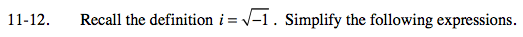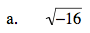4i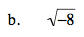$\sqrt{-1}\sqrt{8}$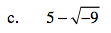$5-\sqrt{-1}\sqrt{9}$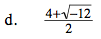$\frac{4}{2}+\frac{\textit{i}\sqrt{12}}{2}$